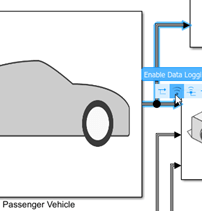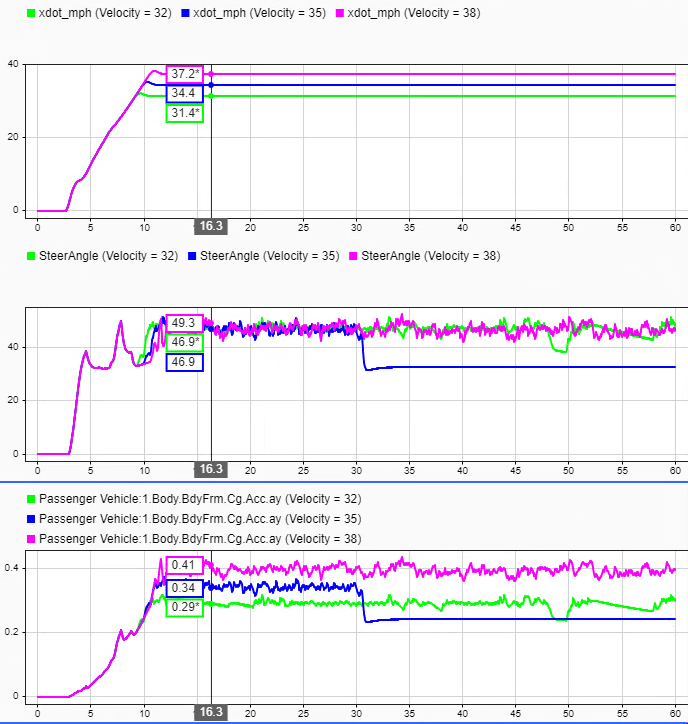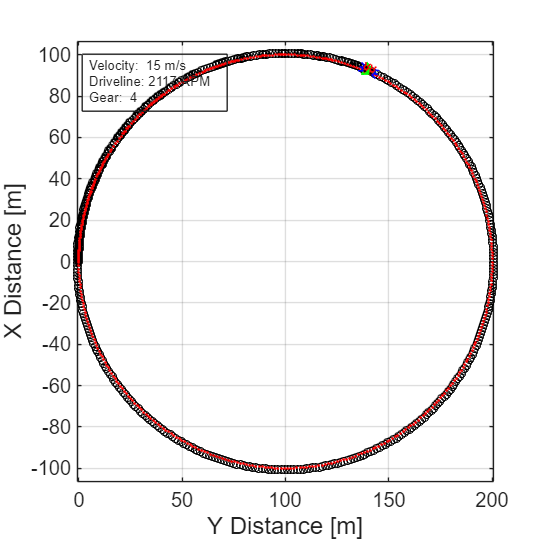# Vehicle Lateral Acceleration at Different Speeds

This example shows how to use the vehicle dynamics constant radius reference application to analyze the impact of speed on the vehicle lateral dynamics. Specifically, you can examine the lateral acceleration when you run the maneuver with different speeds. For information about similar maneuvers, see standards SAE J266_199601 and ISO 4138:2012.

During the maneuver, the vehicle uses a predictive driver model to maintain a pre-specified turn radius at a set velocity.

```helpersetupcr; ```### Run a Constant Radius Maneuver

1. Open the Reference Generator block. By default, the maneuver is set with these parameters:

• Maneuver`Constant radius`

• Use maneuver-specific driver, initial position, and scene — on

• Longitudinal velocity — 35 mph

• Radius value — 100 m

2. Select the Reference Generator block 3D Engine tab. By default, the 3D Engine parameter is Disabled. For the 3D visualization engine platform requirements and hardware recommendations, see the Unreal Engine Simulation Environment Requirements and Limitations.

3. Run the maneuver with the default settings. As the simulation runs, view the vehicle information.

```mdl = 'CRReferenceApplication'; sim(mdl); ```
``` ### Starting serial model reference simulation build. ### Model reference simulation target for Driveline is up to date. ### Model reference simulation target for PassVeh14DOF is up to date. ### Model reference simulation target for SiMappedEngineV is up to date. Build Summary 0 of 3 models built (3 models already up to date) Build duration: 0h 0m 11.132s ```• In the Vehicle Position window, view the vehicle longitudinal distance as a function or the lateral distance. The yellow line displays the yaw rate. The blue line shows the steering angle.

• In the Visualization subsystem, open the Steer, Velocity, Lat Accel Scope block to display the steering angle, velocity, and lateral acceleration versus time.### Sweep Speed

Run the constant radius reference application with three different speeds. Stop the simulation if the vehicle exceeds a lateral acceleration threshold of .5 g.

1. In the slowly increasing steering reference application model CRReferenceApplication, open the Reference Generator block. The Longitudinal speed set point, xdot_r block parameter sets the vehicle speed. By default, the speed is 50 mph.

2. Enable signal logging for the velocity, lane, and ISO signals. You can use the Simulink® editor or, alternatively, these MATLAB® commands. Save the model.

• Select the Reference Generator block Stop simulation at lateral acceleration threshold parameter.```set_param([mdl '/Reference Generator'],'cr_ay_stop','on'); ```
• Enable signal logging for the Reference Generator Vis signal outport.```ph=get_param('CRReferenceApplication/Reference Generator','PortHandles'); set_param(ph.Outport(1),'DataLogging','on'); ```
• Enable signal logging for the Passenger Vehicle block outport signal.```ph=get_param('CRReferenceApplication/Passenger Vehicle','PortHandles'); set_param(ph.Outport(1),'DataLogging','on'); ```
• In the Visualization subsystem, enable signal logging for the ISO block.```set_param([mdl '/Visualization/ISO 15037-1:2006'],'Measurement','Enable'); ```

3. Set up a speed set point vector, xdot_r, that you want to investigate. For example, at the command line, type:

```vmax = [35, 40, 45]; numExperiments = length(vmax); ```

4. Create an array of simulation inputs that set the Reference Generator block parameter Longitudinal velocity reference, xdot_r equal to `xdot_r`.

```for idx = numExperiments:-1:1 in(idx) = Simulink.SimulationInput(mdl); in(idx) = in(idx).setBlockParameter([mdl '/Reference Generator'], ... 'xdot_r', num2str(vmax(idx))); end ```

5. Save the model and run the simulations.

```save_system(mdl) tic; simout = parsim(in,'ShowSimulationManager','on'); toc; ```
```[25-May-2022 11:59:31] Checking for availability of parallel pool... Starting parallel pool (parpool) using the 'Processes' profile ... Connected to the parallel pool (number of workers: 6). [25-May-2022 12:00:58] Starting Simulink on parallel workers... [25-May-2022 12:01:30] Loading project on parallel workers... [25-May-2022 12:01:30] Configuring simulation cache folder on parallel workers... [25-May-2022 12:01:46] Loading model on parallel workers... [25-May-2022 12:02:32] Running simulations... [25-May-2022 12:05:00] Completed 1 of 3 simulation runs [25-May-2022 12:05:01] Completed 2 of 3 simulation runs [25-May-2022 12:05:01] Completed 3 of 3 simulation runs [25-May-2022 12:05:01] Cleaning up parallel workers... Elapsed time is 357.284321 seconds. ```

6. Close the Simulation Data Inspector windows.

### Use Simulation Data Inspector to Analyze Results

Use the Simulation Data Inspector to examine the results. You can use the UI or, alternatively, command-line functions.

1. Open the Simulation Data Inspector. On the Simulink Toolstrip, on the Simulation tab, under Review Results, click Data Inspector.

• In the Simulation Data Inspector, select Import.• In the Import dialog box, clear logsout. Select `simout(1)`, `simout(2)`, and `simout(3)`. Select Import.• Use the Simulation Data Inspector to examine the results.

2. Alternatively, use these MATLAB commands to plot the longitudinal velocity, lateral acceleration, and the steering wheel angle.

```for idx = 1:numExperiments % Create sdi run object simoutRun(idx)=Simulink.sdi.Run.create; simoutRun(idx).Name=['Velocity = ', num2str(vmax(idx))]; add(simoutRun(idx),'vars',simout(idx)); end sigcolor=[0 1 0;0 0 1;1 0 1]; for idx = 1:numExperiments % Extract the lateral acceleration, position, and steering msignal(idx)=getSignalByIndex(simoutRun(idx),268); msignal(idx).LineColor =sigcolor((idx),:); ssignal(idx)=getSignalByIndex(simoutRun(idx),267); ssignal(idx).LineColor =sigcolor((idx),:); asignal(idx)=getSignalByIndex(simoutRun(idx),252); asignal(idx).LineColor =sigcolor((idx),:); end Simulink.sdi.view Simulink.sdi.setSubPlotLayout(3,1); for idx = 1:numExperiments % Plot the lateral position, steering angle, and lateral acceleration plotOnSubPlot(msignal(idx),1,1,true); plotOnSubPlot(ssignal(idx),2,1,true); plotOnSubPlot(asignal(idx),3,1,true); end ```

The results are similar to these plots, which indicate that the greatest lateral acceleration occurs when the vehcile velocity is 45 mph.### Further Analysis

To explore the results further, use these commands to extract the lateral acceleration, steering angle, and vehicle trajectory from the `simout` object.

1. Extract the lateral acceleration and steering angle. Plot the data. The results are similar to this plot.

```figure for idx = 1:numExperiments % Extract Data log = get(simout(idx),'logsout'); sa=log.get('Steering-wheel angle').Values; ay=log.get('Lateral acceleration').Values; firstorderfit = polyfit(sa.Data,ay.Data,1); gain(idx)=firstorderfit(1); legend_labels{idx} = [num2str(vmax(idx)), ' mph: Gain = ', ... num2str(gain(idx)), ' m/(deg s^2)']; % Plot steering angle vs. lateral acceleration plot(sa.Data,ay.Data) hold on end % Add labels to the plots legend(legend_labels, 'Location', 'best'); title('Lateral Acceleration') xlabel('Steering Angle [deg]') ylabel('Acceleration [m/s^2]') grid on ```2. Extract the vehicle path. Plot the data. The results are similar to this plot.

```figure for idx = 1:numExperiments % Extract Data log = get(simout(idx),'logsout'); x = log{1}.Values.Body.InertFrm.Cg.Disp.X.Data; y = log{1}.Values.Body.InertFrm.Cg.Disp.Y.Data; legend_labels{idx} = [num2str(vmax(idx)), ' mph']; % Plot vehicle location axis('equal') plot(y,x) hold on end % Add labels to the plots legend(legend_labels, 'Location', 'best'); title('Vehicle Path') xlabel('Y Position [m]') ylabel('X Position [m]') grid on ```J266_199601. Steady-State Directional Control Test Procedures for Passenger Cars and Light Trucks. Warrendale, PA: SAE International, 1996.

 ISO 4138:2012. Passenger cars -- Steady-state circular driving behaviour -- Open-loop test methods. ISO (International Organization for Standardization), 2012.